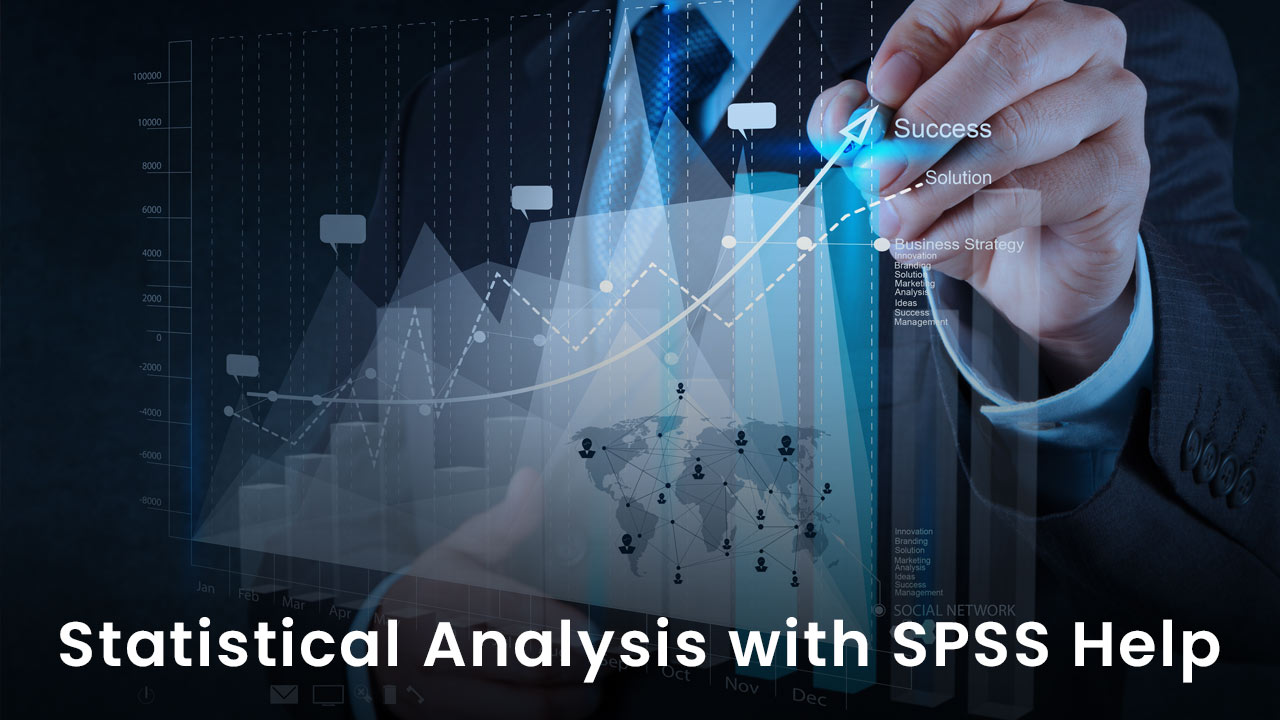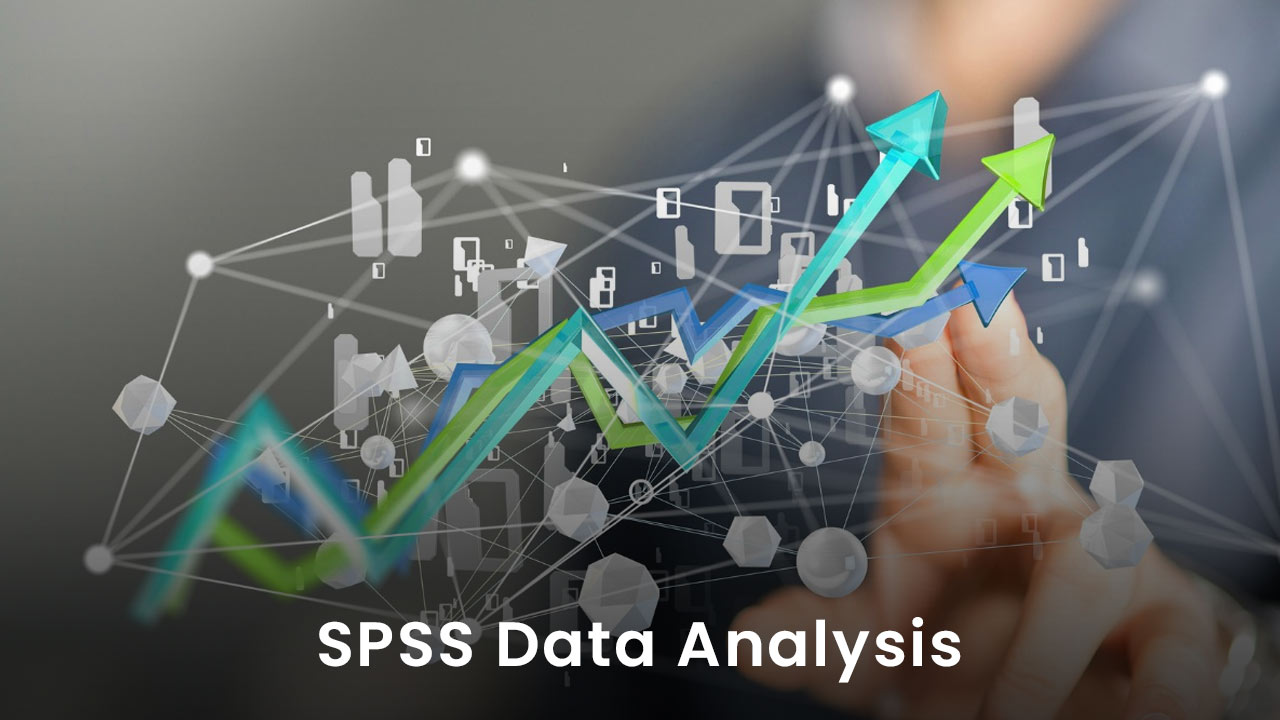# What Is Statistical Analysis and What Are Its Types?## Introduction

Statistical analysis means collecting, interpreting, and analysing the data to understand patterns and trends of a particular data type and develop good decision-making. This type of data analysis is generally used when gathering research data, conducting surveys & studies and collecting a large volume of information, and for business intelligence organisations which work with big data structures. Analysts or researchers take SPSS help to perform this analysis.
The main purpose of statistical analysis is to collect a large volume of data and analyse it. To draw relevant conclusions from big data volumes, the data needs to be computed and statistical analysis deduces information from it and represents it in the form of graphs, charts, tables, etc. Here we will learn about the types of statistical analysis in detail.

## Types of statistical analysis

1. ### Descriptive statistical analysis:

As its name suggests, the descriptive statistical analysis provides descriptions of the data. It condenses all the details and particulars of the collected data so that a thorough meaning can be obtained from the interpretation. To drive the research to a necessary conclusion and to get elaborated quantitative descriptions of the gathered data, descriptive statistical analysis is used. The researchers prefer to take help with SPSS to conduct descriptive statistical analysis in a better way.

2. ### Inferential statistical analysis:

The inferential statistical analysis offers a generalisation of the huge data through the mode of data sampling. Here the researchers represent the big data through a sample set of data using estimated parameters or testing hypothesis methods.

3. ### Predictive statistical analysis:

The predictive statistical analysis makes predictions about the data based on previous regular events. A series of events is being analysed under this type of analysis so that future events and possibilities can be predicted and necessary conclusions can be drawn. Because of the nature of the data analysis technique, this method is used in every sphere of life. Since this technique uses algorithms, simulations, graph analysis, and much more hence it helps in making decisions, shows effectiveness, and provides solutions to future issues. Researchers and students use this statistical analysis technique to predict uncertainties and risks involved in their studies.

4. ### Prescriptive statistical analysis:

The prescriptive statistical analysis tries to provide precision about a future event that what will happen and when will it happen. Based on data mining and artificial intelligence, this statistical analysis combines the information from internal sources and the information from third-party sources. Doing so helps this technique to get insights for developing better possibilities for future events and operations. Since this statistical analysis technique is capable of predicting the future, therefore it describes the ideas to gain the best out of all the consequences.

5. ### Casual statistical analysis:

Researchers use this casual statistical analysis technique to find the reasons and causes of a particular event happening that way. This technique tries to find out why an event is taking place in a particular manner and what will be its outcome; success or failure. To resist or prevent disasters at a larger rate, this statistical method is used.

6. ### Exploratory data analysis:

The exploratory data analysis explores distinct sets of data and then summarises them to get relevant information to the research questions. The researchers use this statistical analysis method to identify hidden patterns in the collected information and then they express these derivations through graphical or non-graphical methods.

##Conclusion

Different types of statistical analysis techniques are used in different situations on various kinds of information. But the core objective for all of these analysis techniques is the same, that is, derive results from the collected information so that better circumstances can be generated in future. SPSS data analysis performed by the experts is the best analysis practice to conduct research effectively. Researchers prefer to adopt this methodology of analysis because of its productive outcomes. Hence, choose a type of statistical analysis as per the type of your study, information, and data structure. Good luck with your statistical analysis!